Homework Help Question & Answers

# Calculate the molar solubility, S, of Ag2(Cros) in a 0.414 MK (CrO4) solution. Note that K2...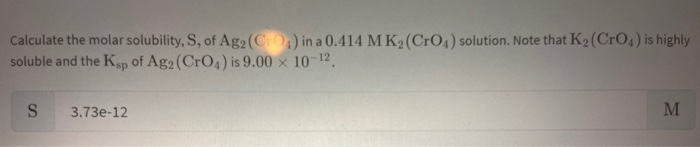Calculate the molar solubility, S, of Ag2(Cros) in a 0.414 MK (CrO4) solution. Note that K2 (Cro) is highly soluble and the Ksp of Ag2 (CrO ) is 9.00 x 10-12 S 3.73e-12

#### Homework Answers

Answer #1

Dissociation of Ag2CrO4 can be represented as

Ag2CrO4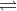2Ag++CrO4-2

2S S where S=solubility

solubility product of  Ag2CrO4 ,Ksp=9.00 x 10-12

K2CrO4 Dissociates to give K+ and CrO4-2 ions

K2CrO4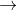2 K++ CrO4-2

initial concentration 0.414M 0 0

change 0.414 - 0.414 0+2x0.414 0+0.414

final concentration 0 0.828 0.414

solubility of Ag2CrO4 will be decreased when  K2CrO4 is added .it is due to common ion effect .common ion is

CrO4-2

when K2CrO4 is added

Ag2CrO42Ag++CrO4-2

2S S

S+0.414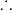[CrO4-2 ] = S+0.414 M

Ksp= [Ag+]2[CrO4-2 ] =  9.00 x 10-12​​​​​​​

9.00 x 10-12​​​​​​​ = S X [S+0.414] Solubility,S=   9.00 x 10-12​​​​​​​ / [S+0.414]

=9.00 x 10-12​​​​​​​ / 0.414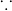S<<<< 0.414

Solubility,S= 2.1739 X 10-11 M

Know the answer?
Your Answer:

#### Post as a guest

Your Name:

What's your source?

#### Earn Coin

Coins can be redeemed for fabulous gifts.

Not the answer you're looking for? Ask your own homework help question. Our experts will answer your question WITHIN MINUTES for Free.
Similar Homework Help Questions
• ### please andwer all qeustions (24) Ag2 S (s) has the solubility equilibrium in water solution: Ag2...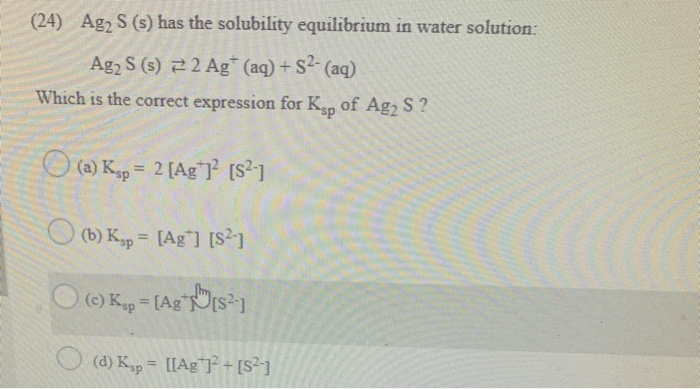please andwer all qeustions (24) Ag2 S (s) has the solubility equilibrium in water solution: Ag2 S (s) 22 Ag+ (aq) + S2- (aq) Which is the correct expression for Ksp of Ag2 S ? (a) Ksp = 2 [Ag 1? [S2-1 (b) Kyp = [Ag+] [S?) (C) Kop = [Ag 1824 (d) Ksp = [[Ag 7? + [5243 (25) When dissolving slightly soluble CaCO3 (s) in water, a solubility equilibrium is reached, with (Ca2+] = [CO3 2-1 = 5.3...

• ### Please answer these questions: -Calculate the molar solubility of Cr2(CrO4)3 (Ksp = 6.47 x 10-22) in...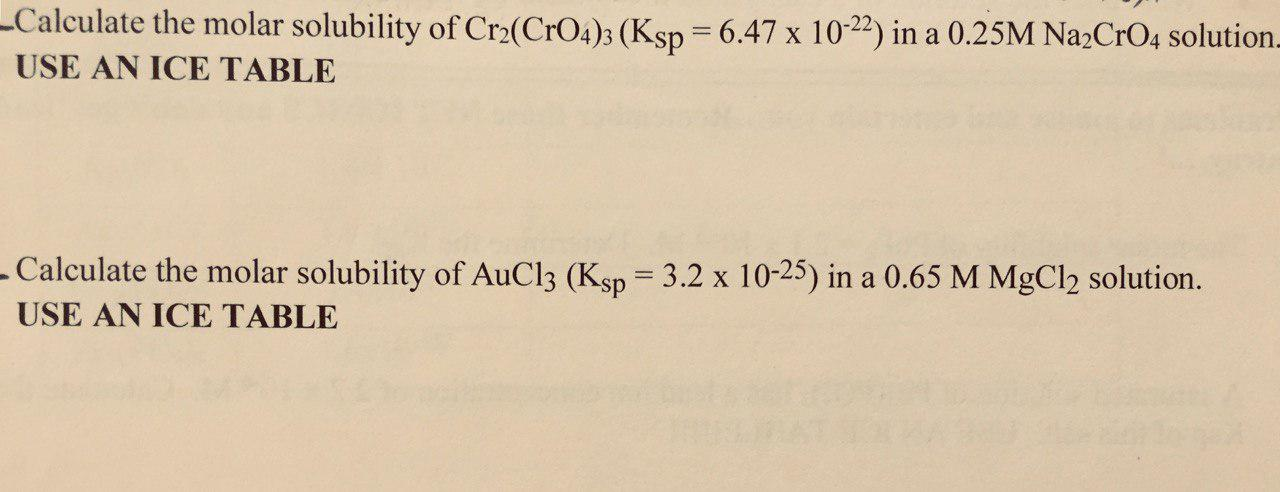Please answer these questions: -Calculate the molar solubility of Cr2(CrO4)3 (Ksp = 6.47 x 10-22) in a 0.25M Na2CrO4 solution. USE AN ICE TABLE Calculate the molar solubility of AuCl3 (Ksp = 3.2 x 10-25) in a 0.65 M MgCl2 solution. USE AN ICE TABLE

• ### Question 20 Silver chromate, Ag Cros, has a Ksp of 8.96 x 10-12 Calculate the molar...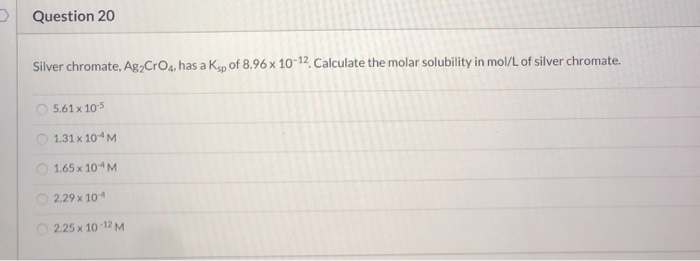Question 20 Silver chromate, Ag Cros, has a Ksp of 8.96 x 10-12 Calculate the molar solubility in mol/L of silver chromate. 5.61 x 105 1.31 x 104M 1.65 x 10'M 2.29 x 104 2.25 x 10-12M

• ### Given the Ksp = 1.2 x 10-12 for Ag2 CrO4 and K+ = 1.7 x 107...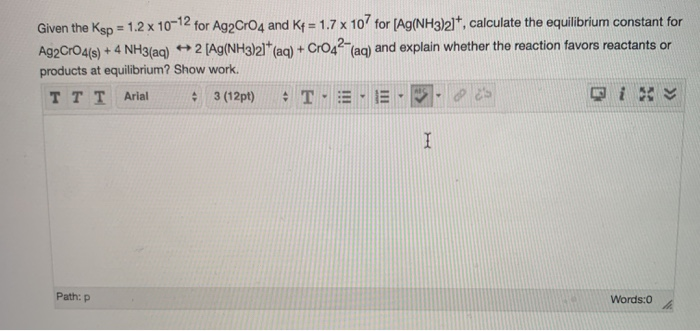Given the Ksp = 1.2 x 10-12 for Ag2 CrO4 and K+ = 1.7 x 107 for (Ag(NH3)21, calculate the equilibrium constant for Ag2CrO4(s) + 4 NH3(aq) + 2 Ag(NH3)21+ (aq) + Cr042-aq) and explain whether the reaction favors reactants or products at equilibrium? Show work. TTT Arial 3 (12pt) • T. E. 3. 's Path:p Words:0

• ### Part A At 25°C, Ksp for Ag2 CrO, is 1.1 x 10-12. Calculate the standard free-energy...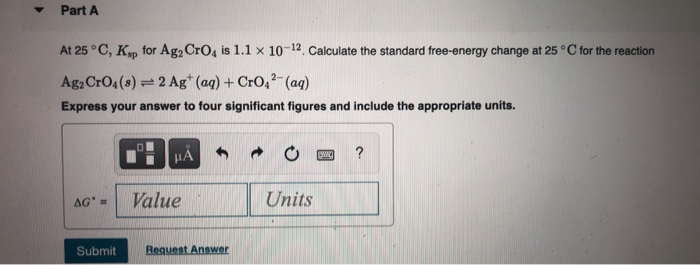Part A At 25°C, Ksp for Ag2 CrO, is 1.1 x 10-12. Calculate the standard free-energy change at 25°C for the reaction Ag2 CrO4(s) = 2 Ag+ (aq) + CrO42- (aq) Express your answer to four significant figures and include the appropriate units. 0 | ЦА wy ? AG - Value Units Submit Request Answer Part A Under which of the following conditions would one mole of Ne have the highest entropy, S? O 131ºC and 21 L O 28°C...

• ### Answer the following questions regarding molar solubility and Ksp. a.) Calculate the molar solubility of Pbly...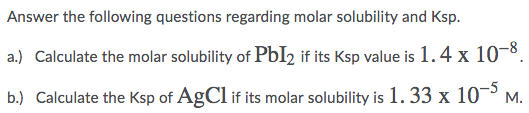Answer the following questions regarding molar solubility and Ksp. a.) Calculate the molar solubility of Pbly if its Ksp value is 1.4 x 10-8. b.) Calculate the Ksp of AgCl if its molar solubility is 1.33 x 10-5 M.

• ### Calculate the molar solubility of Mg(OH)2 in a solution that is basic with a pH of...

Calculate the molar solubility of Mg(OH)2 in a solution that is basic with a pH of 12.62. Ksp = [Mg2+][OH–]2 = 5.6 × 10–12

• ### determine the molar solubility (S) of Ag2CO3 in a buffered solution with a pH of 3.519...

determine the molar solubility (S) of Ag2CO3 in a buffered solution with a pH of 3.519 using the systematic treatment of equilibrium. Ksp (Ag2CO3) = 8.46x10^-12 ; Ka1 (H2CO3) = 4.45x10^-7 ; Ka2 (H2CO3) = 4.69 x 10^-11

• ### □ Resources Hint 597/1400 Check Ans nt Score 10 of14 > Determine the molar solubility (S) of Ag CO, in a buffered solution with a pH of 3.036 using the systematic treatment of equilibrium. Ksp...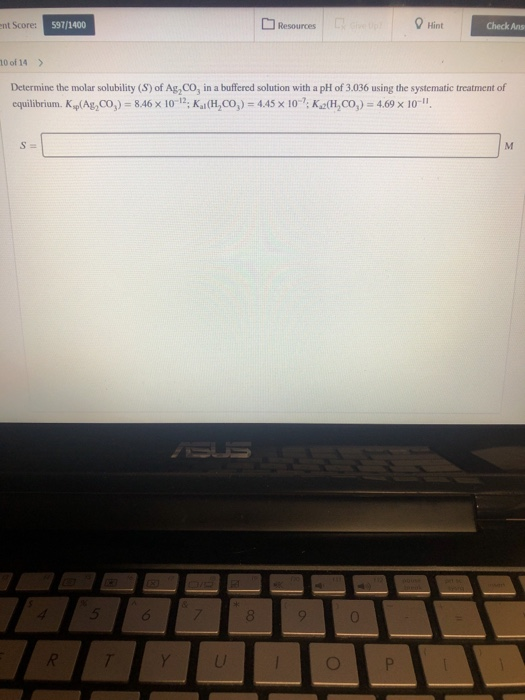□ Resources Hint 597/1400 Check Ans nt Score 10 of14 > Determine the molar solubility (S) of Ag CO, in a buffered solution with a pH of 3.036 using the systematic treatment of equilibrium. Ksp(Ag2CO): 8.46 × 10-12. KadH2CO) = 4.45 × 10-7. K2(H2CO): 4.69 × 10-I, 8 □ Resources Hint 597/1400 Check Ans nt Score 10 of14 > Determine the molar solubility (S) of Ag CO, in a buffered solution with a pH of 3.036 using the systematic treatment...

• ### JIT (Ships Odt a References Calculate the molar solubility of Au Br in 0.015M KBr solution....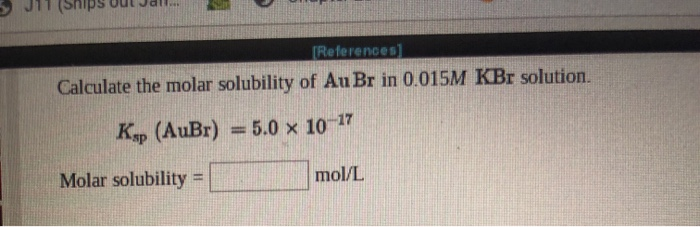JIT (Ships Odt a References Calculate the molar solubility of Au Br in 0.015M KBr solution. 5.0 x 10-17 Ksp (AuBr) Molar solubility mol/L

Free Homework App

Scan Your Homework
to Get Instant Free Answers
Need Online Homework Help?

Get Answers For Free
Most questions answered within 3 hours.